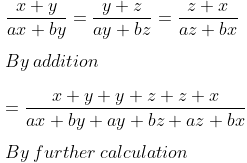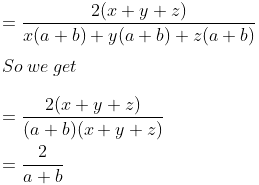Guru

# If x+y/ax+by=y+z/ay+bz=z+x/az+bx , prove that each of these ratio is equal to 2/a+b unless x + y + z = 0

• 0

exam oriented an important question from ML aggarwal, class 10th, chapter 7, ratio and proportion, Avichal publication

In this question ques we have been given that

x+y/ax+by=y+z/ay+bz=z+x/az+bx ,

We have to prove that each of these ratio is equal to 2/a+b unless x + y + z = 0

Question 19, 7.3

Share

1. Solution:

It is given thatIf x + y + z ≠ 0

Therefore, it is proved.

• 0